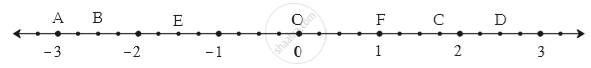SSC (English Medium) Class 8Maharashtra State Board
Share

# Observe the Number Line and Answer the Questions. - SSC (English Medium) Class 8 - Mathematics

ConceptTo Show Rational Numbers on a Number Line

#### Question

Observe the number line and answer the questions.(1) Which number is indicated by point B?

(2) Which point indicates the number 1 3/4 ?

(3) State whether the statement, ‘the point D denotes the number 5/2’ is true or false.

#### Solution

(1) We observe that each unit on the number line is divided into 4 equal parts.
Now, B is the tenth point on the left of 0.
So , B indicates - 10/4 on the number line.

(2) 1 3/4 = 7/4 = 7 xx 1/4
So , the  seventh point on the right of 0 is C that indicates 1 3/4 on the number line.

(3) The point D is the tenth point on the right of 0. So, D indicates 10/4 on the number line.

Now , 10/4 = 5/2

So , D denotes 5/2 on the number line . Hence , the given statement is true .

Is there an error in this question or solution?

#### APPEARS IN

Balbharati Solution for Balbharati Class 8 Mathematics (2019 to Current)
Chapter 1: Rational and Irrational numbers
Practice Set 1.1 | Q: 2 | Page no. 2
Solution Observe the Number Line and Answer the Questions. Concept: To Show Rational Numbers on a Number Line.
S